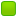###Author Topic: Problems  (Read 2598 times)

0 Members and 1 Guest are viewing this topic.

####Ender

• Moderator
• Hero Member
••• Posts: 2398##### Problems
« on: February 27, 2008, 06:21:19 AM »
I will post problems here when I feel like it.

####Ender

• Moderator
• Hero Member
••• Posts: 2398##### Re: Problems
« Reply #1 on: February 27, 2008, 06:24:39 AM »
Problem 1. Source: aops

A square and equilateral triangle are circumscribed around a common circle. What is the ratio of their areas?

####rabbit

• x86
• Hero Member
••• Posts: 8099
• I speak for the entire clan (except Joe)##### Re: Problems
« Reply #2 on: February 27, 2008, 08:09:32 AM »
Jew!  Wait...

####d&q

• Hero Member
••• Posts: 1429
• I'm here.##### Re: Problems
« Reply #3 on: February 27, 2008, 09:13:00 PM »
Since the triangle is equilateral, the center of the circle is not only the incenter but the centroid. Therefore, the height of the triangle is in a 3:1 ratio with the radius of the circle. Assuming a unit circle, the height is 3, and the area is 3*sqrt(3). The square's area is 1.

Triangle:Square = 3sqrt(3)/4.

: Haha fuck, the squares area is 4, lulz.
« Last Edit: February 27, 2008, 09:15:57 PM by Deuce »
The writ of the founders must endure.

####Ender

• Moderator
• Hero Member
••• Posts: 2398##### Re: Problems
« Reply #4 on: February 28, 2008, 03:41:30 AM »
Since the triangle is equilateral, the center of the circle is not only the incenter but the centroid. Therefore, the height of the triangle is in a 3:1 ratio with the radius of the circle. Assuming a unit circle, the height is 3, and the area is 3*sqrt(3). The square's area is 1.

Triangle:Square = 3sqrt(3)/4.

: Haha fuck, the squares area is 4, lulz.

Correct!